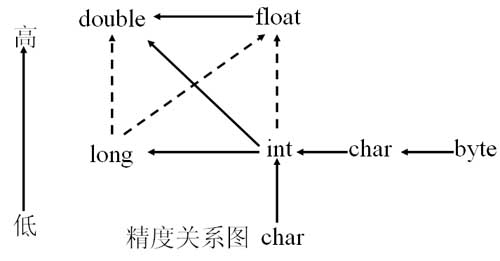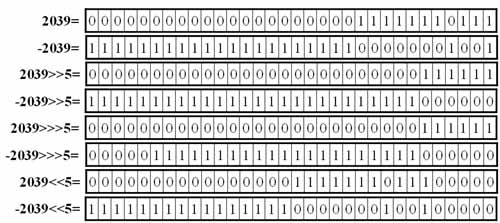# Java的位运算符详解实例——与（&）、非（~）、或（|）、异或（^）

1．与运算符

public class data13
{
public static void main(String[] args)
{
int a=129;
int b=128;
System.out.println("a 和b 与的结果是："+(a&b));
}
}

a 和b 与的结果是：128

“a”的值是129，转换成二进制就是10000001，而“b”的值是128，转换成二进制就是10000000。根据与运算符的运算规律，只有两个位都是1，结果才是1，可以知道结果就是10000000，即128。

2．或运算符

public class data14
{
public static void main(String[] args)
{
int a=129;
int b=128;
System.out.println("a 和b 或的结果是："+(a|b));
}
}

a 和b 或的结果是：129

a 的值是129，转换成二进制就是10000001，而b 的值是128，转换成二进制就是10000000，根据或运算符的运算规律，只有两个位有一个是1，结果才是1，可以知道结果就是10000001，即129。

3．非运算符

public class data15
{
public static void main(String[] args)
{
int a=2;
System.out.println("a 非的结果是："+(~a));
}
}

4．异或运算符

public class data16
{
public static void main(String[] args)
{
int a=15;
int b=2;
System.out.println("a 与 b 异或的结果是："+(a^b));
}
}

a 与 b 异或的结果是：13

Java中的运算符（操作符）

程序的基本功能是处理数据，任何编程语言都有自己的运算符。因为有了运算符，程序员才写出表达式，实现各种运算操作，实现各种逻辑要求。

为实现逻辑和运算要求，编程语言设置了各种不同的运算符，且有优先级顺序，所以有的初学者使用复杂表达式的时候搞不清楚。这里详细介绍一下Java中的运算符。

Java运算符很多，下面按优先顺序列出了各种运算符。

 优先级 运算符分类 结合顺序 运算符 由 高 到 低 分隔符 左结合 .    []     ( )     ;      , 一元运算符 右结合 !  ++     --     -   ~ 算术运算符 移位运算符 左结合 *     /      %    +     -      <<   >>   >>> 关系运算符 左结合 <     >     <=   >=   instanceof(Java 特有)   = =  != 逻辑运算符 左结合 ! &&  ||  ~  &  |  ^ 三目运算符 右结合 布尔表达式?表达式1:表达式2 赋值运算符 右结合 =  *=     /=  %=   +=   -=    <<= >>= >>>=  &=  *=  |=

因操作数是一个，故称为一元运算符。

 运算符 含义 例子 - 改变数值的符号，取反 -x（-1*x） ~ 逐位取反，属于位运算符 ~x ++ 自加1 x++ -- 自减1 x--

++x 因为++在前，所以先加后用。
x++ 因为++在后，所以先用后加。

int a=10;
int b=10;
int sum=a+ ++b;
System.out.println("a="+a+",b="+b+",sum="+sum);

int a=10;
int b=10;
int sum=a+++b;
System.out.println("a="+a+",b="+b+",sum="+sum);

n=10;
m=~n;

所谓算术运算符，就是数学中的加、减、乘、除等运算。因算术运算符是运算两个操作符，故又称为二元运算符。

 运算符 含义 例子 + 加法运算 x+y - 减法运算 x-y * 乘法运算 x*y / 除法运算 x/y % 取模运算（求余运算） x%y

这些操作可以对不同类型的数字进行混合运算，为了保证操作的精度，系统在运算过程中会做相应的转化。数字精度的问题，我们在这里不再讨论。下图中展示了运算过程中，数据自动向上造型的原则。注：1、实线箭头表示没有信息丢失的转换，也就是安全性的转换，虚线的箭头表示有精度损失的转化，也就是不安全的。
2、当两个操作数类型不相同时，操作数在运算前会子松向上造型成相同的类型，再进行运算。

1. int a=22;
2. int b=5;
3. double c=5;
4.
5. System.out.println(b+"+"+c+"="+(b+c));
6. System.out.println(b+"-"+c+"="+(b-c));
7. System.out.println(b+"*"+c+"="+(b*c));
8. System.out.println(a+"/"+b+"="+(a/b));
9. System.out.println(a+"%"+b+"="+(a%b));
10. System.out.println(a+"/"+c+"="+(a/c));
11. System.out.println(a+"%"+c+"="+(a%c));

5+5.0=10.0
5-5.0=0.0
5*5.0=25.0
22/5=4
22%5=2
22/5.0=4.4
22%5.0=2.0

移位运算符操作的对象就是二进制的位，可以单独用移位运算符来处理int型整数。

 运算符 含义 例子 << 左移运算符，将运算符左边的对象向左移动运算符右边指定的位数（在低位补0） x<<3 >> "有符号"右移运算 符，将运算符左边的对象向右移动运算符右边指定的位数。使用符号扩展机制，也就是说，如果值为正，则在高位补0，如果值为负，则在高位补1. x>>3 >>> "无符号"右移运算 符，将运算符左边的对象向右移动运算符右边指定的位数。采用0扩展机制，也就是说，无论值的正负，都在高位补0. x>>>31. System.out.println(Integer.toBinaryString(6297));
2. System.out.println(Integer.toBinaryString(-6297));
3. System.out.println(Integer.toBinaryString(6297>>5));
4. System.out.println(Integer.toBinaryString(-6297>>5));
5. System.out.println(Integer.toBinaryString(6297>>>5));
6. System.out.println(Integer.toBinaryString(-6297>>>5));
7. System.out.println(Integer.toBinaryString(6297<<5));
8. System.out.println(Integer.toBinaryString(-6297<<5));

运行结果：

1100010011001
11111111111111111110011101100111
11000100
11111111111111111111111100111011
11000100
111111111111111111100111011
110001001100100000
11111111111111001110110011100000

从计算速度上讲，移位运算要比算术运算快。
如果x是负数，那么x>>>3没有什么算术意义，只有逻辑意义。

Java具有完备的关系运算符，这些关系运算符同数学中的关系运算符是一致的。具体说明如下：

 运算符 含义 例子 < 小于 x 大于 x>y <= 小于等于 x<=y >= 大于等于 x>=y == 等于 x==y != 不等于 x!=y

instanceof操作符用于判断一个引用类型所引用的对象是否是一个类的实例。操作符左边的操作元是一个引用类型，右边的操作元是一个类名或者接口，形式如下：

obj instanceof ClassName      或者    obj instanceof InterfaceName

 A !A true false false true

 A B A&&B false false false true false false false true false true true true

 A B A||B false false false true false true false true true true true true

if(1==1 && 1==2 && 1==3){  }

“短路”现象在多重判断和逻辑处理中非常有用。我们经常这样使用：

1. public void a(String str){
2.   if(str!=null && str.trim().length()>0){
3.
4.   }
5. }

 A ~A 1 0 0 1

 A B A&B 1 1 1 1 0 0 0 1 0 0 0 0

 A B A | B 1 1 1 1 0 1 0 1 1 0 0 0

 A B A&B 1 1 0 1 0 1 0 1 1 0 0 0

1. int a=15;
2. int b=2;
3.
4. System.out.println(a+"&"+b+"="+(a&b));
5. System.out.println(a+"|"+b+"="+(a|b));
6. System.out.println(a+"^"+b+"="+(a^b));

15&2=2
15|2=15
15^2=13

 a 1 1 1 1 15 b 0 0 1 0 2 a&b 0 0 1 0 2 a|b 1 1 1 1 15 a^b 1 1 0 1 13

三目运算符是一个特殊的运算符，它的语法形式如下：

int sum=90;
String str=sum<100 ? "失败" : "成功";

String str=null;
if(num<100){
str="失败";
}else{
str="成功";
}

 运算符 例子 含义 += x+=y x=x+y -= x-=y x=x-y *= x*=y x=x*y /= x/=y x=x/y %= x%=y x=x%y >>= x>>=y x=x>>y >>>= a>>>=y x=x>>>y <<= a<<=y x=x<

1. package com.zf.binary;
2.
3.
4. public class Test1 {
5.
6.     public static void main(String[] args) {
7.
8.         /* 符号为:最高位同时表示图号，0为正数，1为负数 */
9.
10.         /*
11.             1、二进制转换为十进制
12.
13.             二进制转换为10进制的规律为： 每位的值 * 2的（当前位-1次方）
14.             例如：
15.                 00000001 = 0 * 2^7 + 0 * 2^6 + 0 * 2^5 + 0 * 2^4 + 0 * 2^3 + 0 * 2^2 + 0 * 2^1 + 1 * 2^0  = 1
16.                 00000010 = 0 * 2^7 + 0 * 2^6 + 0 * 2^5 + 0 * 2^4 + 0 * 2^3 + 0 * 2^2 + 1 * 2^1 + 0 * 2^0  = 2
17.
18.             2、二进制的符号位：
19.                 最高位表示符号位，0表示正数  ， 1表示负数
20.
21.
22.             3、将二进制负数转换为十进制：先对该二进制数取反，然后加1，再转换为十进制，然后在前面加上负号
23.                 例如： 10101011 最高位为1，所以为负数
24.                    第一步：取反： 01010100
25.                    第二步：加1 ： 01010101
26.                    第三步：转换为10进制：85
27.                    第四步：加上负号： -85
28.                    所以      10101011 转换为十进制为 -85
29.
30.             4、将十进制负数转换为二进制：先得到该十进制负数的绝对值，然后转换为二进制，然后将该二进制取反，然后加1
31.                 例如：-85
32.                 第一步：得到绝对值 85
33.                 第二步：转换为二进制：01010101
34.                 第二步：取反：                10101010
35.                 第三步：加1：                 10101011
36.                 所以，-85转换为二进制为  10101011
37.          */
38.
39.         /*
40.         ~ ‘非’ 运算符是将目标数的进制去反，即0变成1 ，1变成0
41.         2的二进制码为 00000010 ， 它取反为11111101 ，可见取反后结果为负数（二进制负数转换为十进制的步骤为：将二进制去反，然后+1）
42.          将 11111101 转换为10进制 ，第一步去反 得到 00000010 然后 加1 得到 00000011 ，得到的结果为3 ，然后在前面加上负号就可以了
43.          所以结果为-3
44.          */
45.         System.out.println(~2);
46.
47.         /*
48.           ^ 异或 ，计算方式为：两个二进制数的位相同则为0 不同则为1
49.           23转换为二进制为：00010111
50.           12转换为二进制为：00001100
51.                     计算结果为：00011011  =  27
52.          */
53.         System.out.println(23 ^ 12);
54.
55.         /*
56.          & 按位与 ，计算方式为：两个二进制数的位都为1则为1 ，否则为0
57.          1的二进制为 ：00000001
58.          2的二进制为 ：00000010
59.               结果为 :00000000 = 0
60.          */
61.         System.out.println(1&2);
62.
63.         /*
64.           | 按位或 ，计算方式为：两个二进制位有一个为1就为1，否者为0
65.           5 的二进制为：00000101
66.           6 的二进制为：00000110
67.                   结果为：00000111 = 7
68.          */
69.         System.out.println( 5 | 6);
70.
71.
72.         /*
73.           >> 有符号右移位  ，符号左边表示要被移位的数，右边表示需要移的位数，结果为正数则在左边补0，否则补1
74.           3 的二进制为：00000010
75.                 向右移动1位：00000001 = 1
76.          */
77.         System.out.println(3 >> 1);
78.
79.
80.     }
81.
82. }
package com.zf.binary;

public class Test1 {

public static void main(String[] args) {

/* 符号为:最高位同时表示图号，0为正数，1为负数 */

/*
1、二进制转换为十进制

二进制转换为10进制的规律为： 每位的值 * 2的（当前位-1次方）
例如：
00000001 = 0 * 2^7 + 0 * 2^6 + 0 * 2^5 + 0 * 2^4 + 0 * 2^3 + 0 * 2^2 + 0 * 2^1 + 1 * 2^0  = 1
00000010 = 0 * 2^7 + 0 * 2^6 + 0 * 2^5 + 0 * 2^4 + 0 * 2^3 + 0 * 2^2 + 1 * 2^1 + 0 * 2^0  = 2

2、二进制的符号位：
最高位表示符号位，0表示正数  ， 1表示负数

3、将二进制负数转换为十进制：先对该二进制数取反，然后加1，再转换为十进制，然后在前面加上负号
例如： 10101011 最高位为1，所以为负数
第一步：取反： 01010100
第二步：加1 ： 01010101
第三步：转换为10进制：85
第四步：加上负号： -85
所以      10101011 转换为十进制为 -85

4、将十进制负数转换为二进制：先得到该十进制负数的绝对值，然后转换为二进制，然后将该二进制取反，然后加1
例如：-85
第一步：得到绝对值 85
第二步：转换为二进制：01010101
第二步：取反：                10101010
第三步：加1：                 10101011
所以，-85转换为二进制为  10101011
*/

/*
~ ‘非’ 运算符是将目标数的进制去反，即0变成1 ，1变成0
2的二进制码为 00000010 ， 它取反为11111101 ，可见取反后结果为负数（二进制负数转换为十进制的步骤为：将二进制去反，然后+1）
将 11111101 转换为10进制 ，第一步去反 得到 00000010 然后 加1 得到 00000011 ，得到的结果为3 ，然后在前面加上负号就可以了
所以结果为-3
*/
System.out.println(~2);

/*
^ 异或 ，计算方式为：两个二进制数的位相同则为0 不同则为1
23转换为二进制为：00010111
12转换为二进制为：00001100
计算结果为：00011011  =  27
*/
System.out.println(23 ^ 12);

/*
& 按位与 ，计算方式为：两个二进制数的位都为1则为1 ，否则为0
1的二进制为 ：00000001
2的二进制为 ：00000010
结果为 :00000000 = 0
*/
System.out.println(1&2);

/*
| 按位或 ，计算方式为：两个二进制位有一个为1就为1，否者为0
5 的二进制为：00000101
6 的二进制为：00000110
结果为：00000111 = 7
*/
System.out.println( 5 | 6);

/*
>> 有符号右移位  ，符号左边表示要被移位的数，右边表示需要移的位数，结果为正数则在左边补0，否则补1
3 的二进制为：00000010
向右移动1位：00000001 = 1
*/
System.out.println(3 >> 1);

}

}


07-304万+07-051万+
03-072589
04-266331
10-184998
03-18957
12-272005
02-121499
07-101万+
08-20
01-241万+
08-2311万+
12-1842万+
02-15